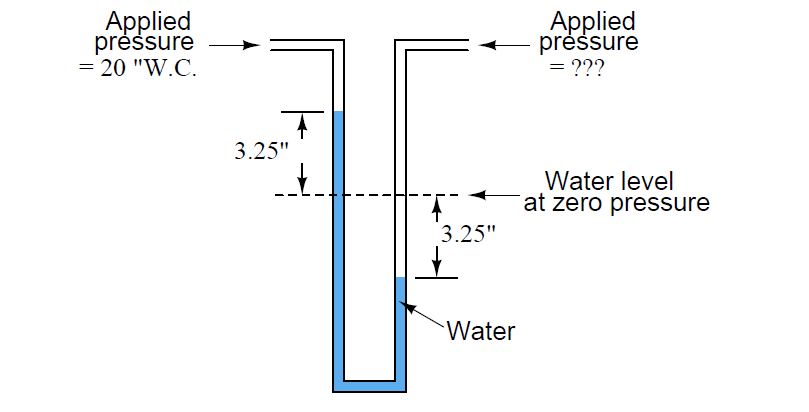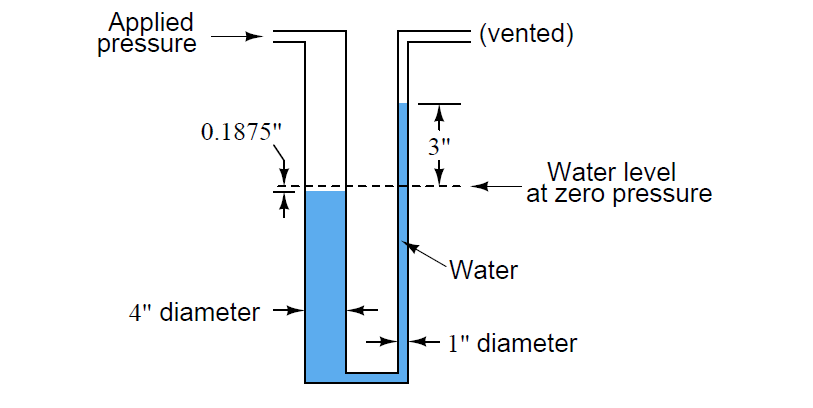# How much Pressure applied to U-tube Water Manometer?

## U-tube Water Manometer

#### Question 1:

How much pressure is being applied to this U-tube water manometer, in units of “inches of water column” and “pounds per square inch”?What would happen to the liquid levels if the water were replaced by an oil with a lesser density? Given the same applied pressure, would the distance between the two liquid columns be greater, less, or the same as shown in the above illustration?

Applied pressure = 9 ”W.C., which is equal to 0.32514 PSI.

For the same applied pressure, the distance between the two liquid columns will be greater than with water. In other words, for a pressure of 9” W.C., there will be more than 9 inches of vertical distance separating the two liquid columns.

Essentially, manometers work on the principle of balanced pressures: the applied gas pressure forces the liquid columns to shift height. When they do so, they generate a hydrostatic pressure proportional to their differential height. When this hydrostatic pressure equals the applied pressure, the liquid columns stop moving and a condition of equilibrium is reached.

If a lighter fluid such as oil is used instead of water, a greater height will have to be developed to generate the same amount of hydrostatic pressure to oppose the applied gas pressure and reach equilibrium. Conversely, if a heavier (denser) liquid such as mercury were to be used, a much smaller vertical height would develop between the two columns for the same pressure.

#### Question 2:

How much pressure, in units of “inches of water column,” is being applied to the right-hand tube of this U-tube water manometer?

Also, convert this pressure into units of Pascals.Pressure applied to right-hand tube = 26.5 ”W.C = 6600.8 Pa.

#### Question 3:

How much pressure is being applied to this U-tube water manometer, in units of “inches of water column” and “pounds per square inch”?Applied pressure = 3.1875 ”W.C., which is equal to 0.1152 PSI.

Credits: Tony Kuphaldt